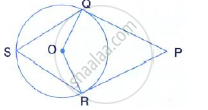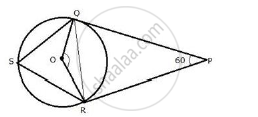Share

# In the Following Figure, Pq and Pr Are Tangents to the Circle, with Centre O. If ∠Qpr = 60°, Calculate: ∠ Qor - Mathematics

Course

#### Question

In the following figure, PQ and PR are tangents to the circle, with centre O. If ∠QPR = 60°, calculate:

∠QOR#### SolutionJoin QR.

OQ  ⊥ OP,OR ⊥  RP
∴ ∠OQP = 90°  ,∠ORP = 90° ,∠QPR = 60°
∠QOR 360° -( 90° + 90° + 60°)
∠QOR = 360° - 240°
∠QOR = 120°

Is there an error in this question or solution?